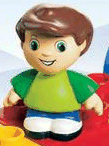# QUATRO Figure

Quatro FigureTheme:
Accessories:

None

Years:
[List of appearances]

The QUATRO Figure is a type of minifigure larger than a DUPLO Figure made from a single piece. There was only one QUATRO figure released in the one set, 5470 My First QUATRO Figure.

LEGO Figures
System Scale: Animal | Big Figure | Fabuland Figure | LEGOLAND Minifigure | Mini-doll figure | Minifigure DUPLO Figure | QUATRO Figure Belville Figure | TECHNIC Figure | Scala Figure | Homemaker Figure Microfigure | Micro Mob | 4+ Figure | Basic figure